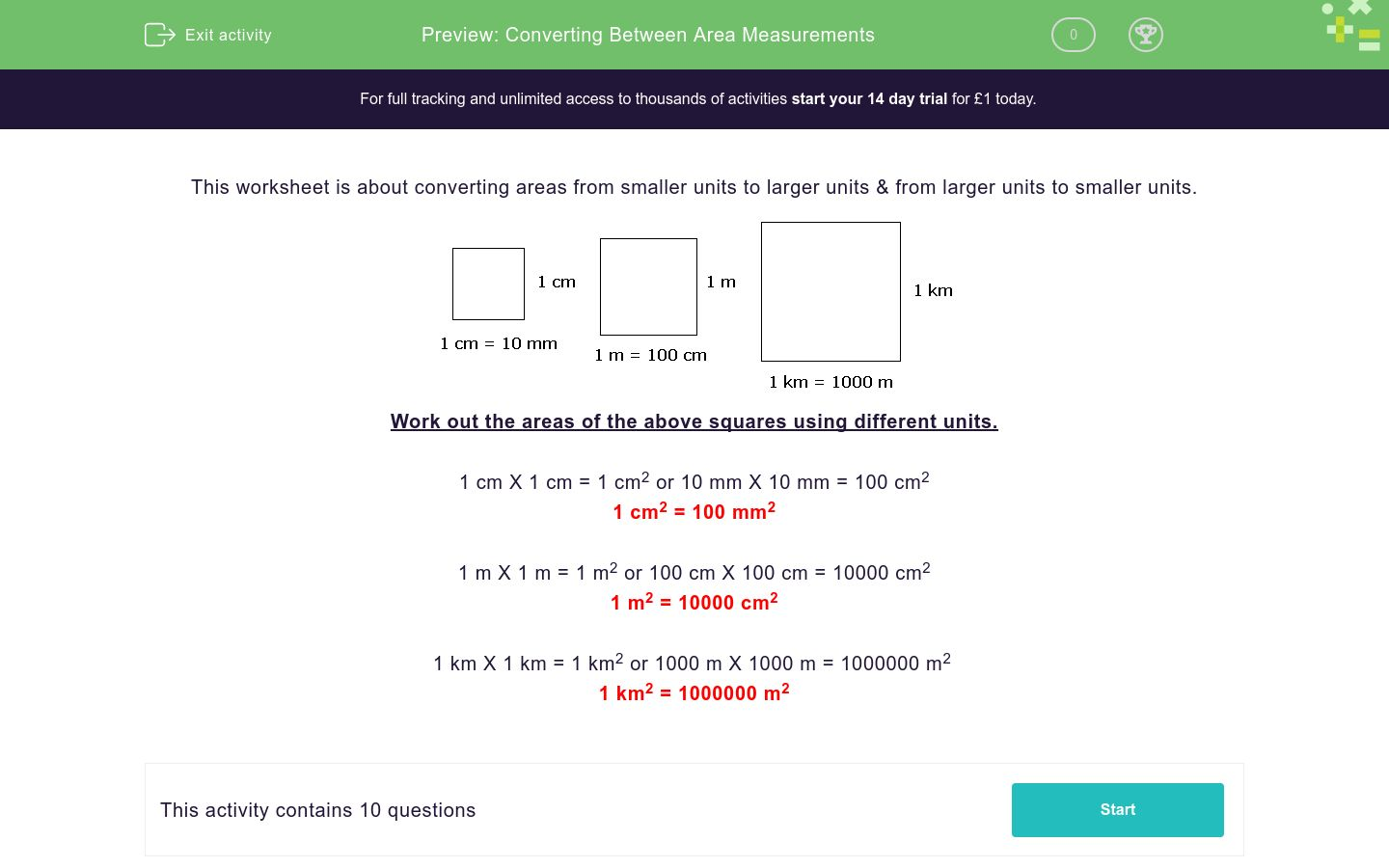# Converting Between Area Measurements

In this worksheet, students convert areas from smaller units to larger units and from larger units to smaller units.Key stage:  KS 3

Curriculum topic:   Ratio, Proportion and Rates of Change

Curriculum subtopic:   Change Between Related Standard Units

Difficulty level:### QUESTION 1 of 10

This worksheet is about converting areas from smaller units to larger units & from larger units to smaller units.Work out the areas of the above squares using different units.

1 cm X 1 cm = 1 cm2 or 10 mm X 10 mm = 100 cm2

1 cm2 = 100 mm2

1 m X 1 m = 1 m2 or 100 cm X 100 cm = 10000 cm2

1 m2 = 10000 cm2

1 km X 1 km = 1 km2 or 1000 m X 1000 m = 1000000 m

1 km2 = 1000000 m2

Convert the following area into the larger unit.

291491.8 cm2 =  m2

Convert the following area into the larger unit.

915625.2 m2 =  km2

Convert the following area into the smaller unit.

67.3 cm2 =  mm2

Convert the following area into the smaller unit.

33.7 m2 =  cm2

Convert the following area into the smaller unit.

74.5 km2 =  m2

Convert the following area into the smaller unit.

95.6 m2 =  mm2

Convert the following area into the smaller unit.

72.9 cm2 =  mm2

Convert the following area into the smaller unit.

12.6 m2 =  cm2

Convert the following area into the smaller unit.

8.8 cm2 =  mm2

Convert the following area into the smaller unit.

73 m2 =  cm2
• Question 1

Convert the following area into the larger unit.

291491.8 cm2 =  m2
29.14918
EDDIE SAYS
There are 100 x 100 = 10000 cm2 in 1m2
• Question 2

Convert the following area into the larger unit.

915625.2 m2 =  km2
0.9156252
EDDIE SAYS
There are 1000 x 1000 = 1000000 m2 in 1km2
• Question 3

Convert the following area into the smaller unit.

67.3 cm2 =  mm2
6730
EDDIE SAYS
There are 10 x 10 = 100 mm2 in 1mm2
• Question 4

Convert the following area into the smaller unit.

33.7 m2 =  cm2
337000
EDDIE SAYS
There are 100 x 100 = 10000 cm2 in 1m2
• Question 5

Convert the following area into the smaller unit.

74.5 km2 =  m2
74500000
EDDIE SAYS
There are 1000 x 1000 = 1000000 m2 in 1km2
• Question 6

Convert the following area into the smaller unit.

95.6 m2 =  mm2
95600000
EDDIE SAYS
There are 1000 x 1000 = 1000000 mm2 in 1m2
• Question 7

Convert the following area into the smaller unit.

72.9 cm2 =  mm2
7290
EDDIE SAYS
There are 10 x 10 = 100 mm2 in 1cm2
• Question 8

Convert the following area into the smaller unit.

12.6 m2 =  cm2
126000
EDDIE SAYS
There are 100 x 100 = 10000 cm2 in 1m2
• Question 9

Convert the following area into the smaller unit.

8.8 cm2 =  mm2
880
EDDIE SAYS
There are 10 x 10 = 100mm2 in 1cm2
• Question 10

Convert the following area into the smaller unit.

73 m2 =  cm2
730000
EDDIE SAYS
There are 100 x 100 = 10000 cm2 in 1m2
---- OR ----

Sign up for a £1 trial so you can track and measure your child's progress on this activity.

### What is EdPlace?

We're your National Curriculum aligned online education content provider helping each child succeed in English, maths and science from year 1 to GCSE. With an EdPlace account you’ll be able to track and measure progress, helping each child achieve their best. We build confidence and attainment by personalising each child’s learning at a level that suits them.

Get started